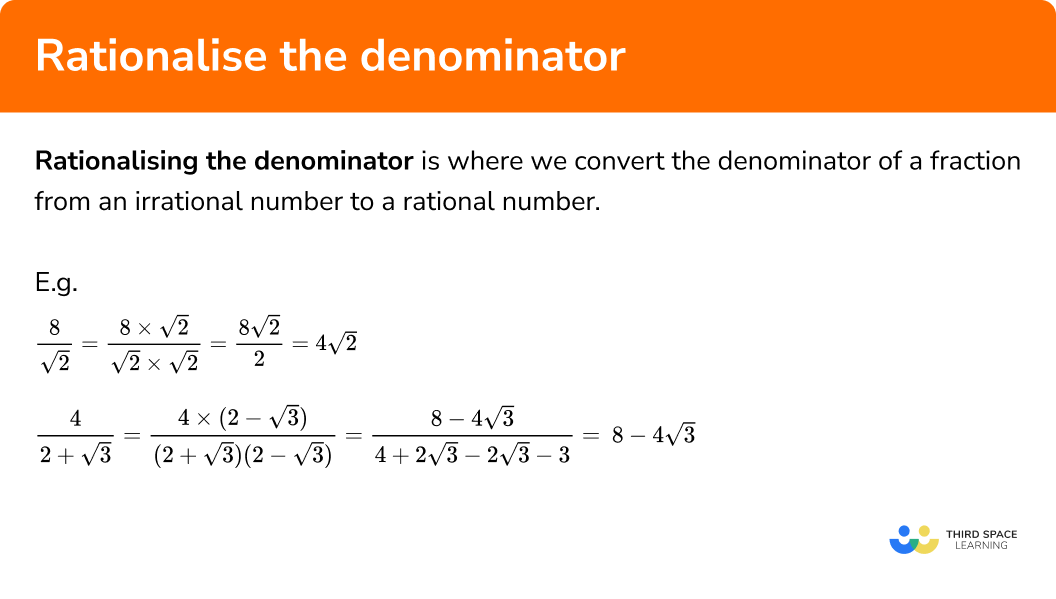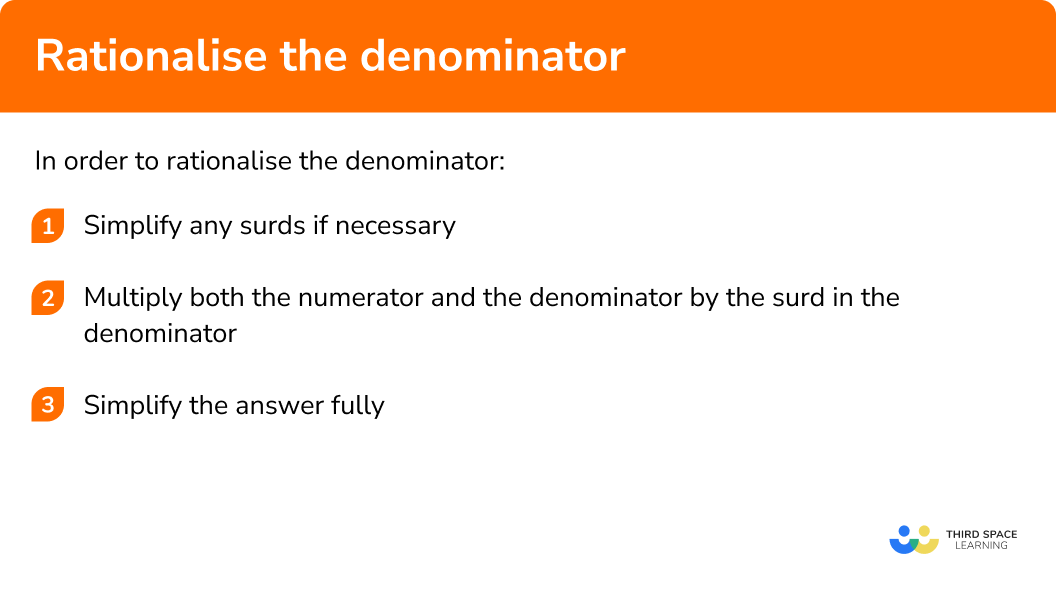GCSE Maths Number Surds

Rationalise The Denominator

# Rationalise the Denominator

Here we will learn about rationalising the denominator of surd expressions including how to rationalise the denominator for simple surd expressions, and then extend these skills to more complicated surd fractions.

There are also rationalising the denominator worksheets based on Edexcel, AQA and OCR exam questions, along with further guidance on where to go next if you’re still stuck.

## What is rationalising the denominator?

Rationalising the denominator is where we convert the denominator of a fraction from an irrational number to a rational number.

E.g.

$\frac{8}{\sqrt{2}}=\frac{8\times\sqrt{2}}{\sqrt{2}\times\sqrt{2}}=\frac{8\sqrt{2}}{2}=4\sqrt{2}$

A number that can be written as an integer (whole number) or a simple fraction is called a rational number.

E.g.

2, 100, −30 are all rational numbers.

So are numbers like \frac{1}{2} , \frac{3}{4} and \frac{1}{9} .

Rational numbers can also be terminating decimals like 0.5 or recurring decimals like 0.111

Any number that can’t be written in this form is called irrational. In decimal form, these are infinite, with no recurring or repeating pattern. Surds are roots which give irrational numbers; remember that at GCSE, we only deal with square root.

All divisions can be written as fractions.

4\div 2 can be written as \frac{4}{2}

In a similar way:

$4 \div \sqrt{2}=\frac{4}{\sqrt{2}}$

However, it’s much easier in mathematics to divide by an integer where possible, so it is useful to be able to convert surd fractions with irrational denominators (surds on the bottom) to fractions with rational denominators.

We do this by using the ideas associated with equivalent fractions:

If the numerator and denominator are both multiplied by the same number or expression, the fraction remains equivalent to the original.

The process of changing the denominator of a fraction to a rational number in this way is called rationalising (or rationalizing) the denominator.

On this page, we will look at cases where the denominator is a single surd.

Step by step guide: Rationalising surds (coming soon)

### What is rationalising the denominator?## How to rationalise the denominator

In order to rationalise the denominator:

1. Simplify any surds, if necessary.
2. Multiply both the numerator and the denominator by the surd in the denominator.

### How to rationalise the denominator## Rationalising the denominator examples

### Example 1: integer on top, surd on the bottom

Rationalise the denominator:

$\frac{4}{\sqrt{2}}$

1. Simplify any surds, if necessary.

In this example this is already done.

2Multiply both the numerator and the denominator by the surd in the denominator.

So here we multiply the top and the bottom of the fraction by root 2:

$\frac{4 \times \sqrt{2}}{\sqrt{2} \times \sqrt{2}}$

Numerator:

$4 \times \sqrt{2}=4 \sqrt{2}$

Denominator:

$\sqrt{2} \times \sqrt{2}=2$

So the full expression becomes:

$\frac{4 \sqrt{2}}{2}$

The denominator is now rationalised, because 2 is a rational number.

$4\div2=2$

So the final answer actually simplifies to

$2 \sqrt{2}$

### Example 2: integer on top, surd on the bottom

Rationalise the denominator:

$\frac{6}{\sqrt{45}}$

Root 45 will simplify:

\begin{aligned} \sqrt{45} &=\sqrt{9 \times 5} \\ &=\sqrt{9} \times \sqrt{5} \\ &=3 \sqrt{5} \end{aligned}

So here we multiply the top and the bottom of the fraction by root 5:

$\frac{6 \times \sqrt{5}}{3 \sqrt{5} \times \sqrt{5}}$

Numerator:

$6\times\sqrt{5}=6\sqrt{5}$

Denominator:

$3 \sqrt{5} \times \sqrt{5}=3 \times 5=15$

So the full expression becomes:

$\frac{6 \sqrt{5}}{15}$

The denominator is now rationalised, because 15 is a rational number.

$6 \div 15=\frac{6}{15}=\frac{2}{5}$

So the final answer simplifies to

$\frac{2 \sqrt{5}}{5}$

### Example 3: surd expression on the top and bottom

Rationalise the denominator:

$\frac{4\sqrt{3}}{\sqrt{7}}$

Both surds are already in their simplest forms.

So here we multiply the top and the bottom of the fraction by root 7:

$\frac{4 \sqrt{3} \times \sqrt{7}}{\sqrt{7} \times \sqrt{7}}$

Numerator:

$4 \sqrt{3} \times \sqrt{7}=4 \sqrt{21}$

Denominator:

$\sqrt{7} \times \sqrt{7}=7$

So the full expression becomes:

$\frac{4 \sqrt{21}}{7}$

The denominator is now rationalised, because 7 is a rational number.

$\frac{4 \sqrt{21}}{7}$

is already in its simplest form; there are no common factors of the integers and there is no square factor of 21 to simplify the root.

### Example 4: surd expression on the top and bottom, some simplification required

Rationalise the denominator:

$\frac{6\sqrt{8}}{\sqrt{3}}$

Root 8 will simplify:

\begin{aligned} 6 \sqrt{8} &=6 \times(\sqrt{4 \times 2}) \\ &=6 \times(\sqrt{4} \times \sqrt{2}) \\ &=6 \times(2 \sqrt{2}) \\ &=12 \sqrt{2} \end{aligned}

So here we multiply the top and the bottom of the fraction by root 3:

$\frac{12 \sqrt{2} \times \sqrt{3}}{\sqrt{3} \times \sqrt{3}}$

Numerator:

$12 \sqrt{2} \times \sqrt{3}=12 \sqrt{6}$

Denominator:

$\sqrt{3} \times \sqrt{3}=3$

So the full expression becomes:

$\frac{12 \sqrt{6}}{3}$

The denominator is now rationalised, because 3 is a rational number.

12÷3=4

So the final answer simplifies to:

4 \sqrt{6}

### Example 5: combining with adding or subtracting surds

Rationalise the denominator:

$\frac{3 \sqrt{12}+\sqrt{108}}{\sqrt{6}}$

Root 12 will simplify:

\begin{aligned} 3 \sqrt{12} &=3 \times(\sqrt{4 \times 3}) \\ &=3 \times(\sqrt{4} \times \sqrt{3}) \\ =& 3 \times(2 \sqrt{3}) \\ =& 6 \sqrt{3} \end{aligned}

Root 108 will also simplify:

\begin{aligned} \sqrt{108} &=\sqrt{36 \times 3} \\ =& \sqrt{36} \times \sqrt{3} \\ =& 6 \sqrt{3} \end{aligned}

So the numerator is:

$6 \sqrt{3}+6 \sqrt{3}$

and because these are like surds, it will simplify to

$12 \sqrt{3}$

So here we multiply the top and the bottom of the fraction by root 6:

$\frac{12 \sqrt{3} \times \sqrt{6}}{\sqrt{6} \times \sqrt{6}}$

Numerator:

$12 \sqrt{3} \times \sqrt{6}=12 \sqrt{18}$

Denominator:

$\sqrt{6} \times \sqrt{6}=6$

So the full expression becomes:

$\frac{12 \sqrt{18}}{3}$

The denominator is now rationalised, because 6 is a rational number.

Root 18 simplifies further:

\begin{aligned} \sqrt{18} &=\sqrt{9 \times 2} \\ &=\sqrt{9} \times \sqrt{2} \\ &=3 \sqrt{2} \end{aligned}

So the expression becomes:

$\frac{12 \times 3 \sqrt{2}}{6}=\frac{36 \sqrt{2}}{6}$

Finally, 36\div6=6 , so the fully simplified answer is:

$6\sqrt{2}$

### Common misconceptions

• Multiplying by the incorrect surd, if there are surds in both numerator and denominator

Always make sure you multiply both top and bottom of the fraction by the surd in the denominator of the fraction.

• On step 3, if dividing integers to simplify, check that the factor you want to divide by is common to all of the integers in the numerator, as well as the denominator.

E.g.

$\frac{9+6 \sqrt{2}}{3}=3-2 \sqrt{2}$

This can be simplified, because 3 is a factor of 3 , 9 and 6 . However, \frac{4+6 \sqrt{2}}{3} cannot be simplified further, because 3 isn’t a factor of 4 .

Rationalise the denominator is part of our series of lessons to support revision on surds. You may find it helpful to start with the main surds lesson for a summary of what to expect, or use the step by step guides below for further detail on individual topics. Other lessons in this series include:

### Practice rationalising the denominator questions

1. Rationalise the denominator \frac{1}{\sqrt{5}}

\frac{5}{\sqrt{5}}\frac{1}{5}\frac{\sqrt{5}}{5}\sqrt{5}Multiply the numerator and denominator by root 5 .

2. Rationalise the denominator \frac{7}{\sqrt{3}}

\frac{7 \sqrt{3}}{3}\frac{\sqrt{3}}{7}\frac{1}{5}\sqrt{21}Multiply the numerator and denominator by root 3 .

3. Rationalise the denominator \frac{20}{\sqrt{40}}

\frac{20 \sqrt{40}}{40}\frac{\sqrt{40}}{2}\sqrt{20}\sqrt{10}Simplify root 40 first, then multiply the top and bottom by root 10 . Alternatively, you could multiply the top and bottom by root 40 , but remember to simplify your answer fully at the end.

4. Rationalise the denominator \frac{5 \sqrt{3}}{\sqrt{10}}

\sqrt{15}\frac{5 \sqrt{30}}{10}\frac{\sqrt{30}}{2}\frac{\sqrt{30}}{5}Multiply the top and bottom by root 10 , using the multiplication rule of surds: \sqrt{3} \times \sqrt{10}=\sqrt{30}

Finally, simplify the integers: \frac{5}{10}=\frac{1}{2}

5. Rationalise the denominator \frac{5}{4-\sqrt{2}}

\frac{20+5 \sqrt{2}}{14}7+5 \sqrt{2}20-5 \sqrt{2}\frac{20+5 \sqrt{2}}{4}Multiply the top and bottom by 4-\sqrt{2} to give: \frac{5(4-\sqrt{2})}{(4+\sqrt{2})(4-\sqrt{2})}

The denominator simplifies to 16-2=14 , and the numerator expands to become 20-5 \sqrt{2}

6. Rationalise the denominator \frac{2 \sqrt{5}}{3-\sqrt{5}}

\frac{6 \sqrt{5}-10}{4}\frac{2}{3}\frac{5+3 \sqrt{5}}{2}\frac{5 \sqrt{5}}{4}Multiply the top and bottom by 3-\sqrt{5} to give: \frac{2\sqrt{5}(3-\sqrt{5})}{(3-\sqrt{5})(3+\sqrt{5})}

The denominator simplifies to 9-5=4 , and the numerator expands to become 6 \sqrt{5}-10 . Divide through by 2 to give the fully simplified final answer.

### Rationalising the denominator GCSE questions

1. Rationalise the denominator: \frac{12}{2\sqrt{2}}

(2 marks)

\frac{12\sqrt{2}}{4} or \frac{6\sqrt{2}}{2}

(1)

3\sqrt{2}

(1)

2. Simplify fully \frac{(2\sqrt{6}-2)(2\sqrt{6}+2)}{\sqrt{10}}

(3 marks)

(2\sqrt{6}-2)(2\sqrt{6}+2)=24+4\sqrt{6}-4\sqrt{6}-4

Any two terms correct

(1)

All four correct

(1)

\frac{20}{\sqrt10}=\frac{20\sqrt{10}}{10}=2\sqrt{10}

(1)

3. Show that \frac{\sqrt{28}-8}{\sqrt{7}+2} can be written as 10-4\sqrt{7}

(5 marks)

\sqrt{28}=2\sqrt{7}

(1)

\frac{(\sqrt{28}-8)(\sqrt{7}-2)}{(\sqrt{7}+2)(\sqrt{7}-2)}

(1)

\frac{30-12\sqrt{7}}{3} ( 1 mark for numerator, 1 mark for denominator)

(2)

10-4\sqrt{7}

(1)

## Learning checklist

You have now learned how to:

• Rationalise the denominator when it is a single surd
• Rationalise the denominator when it contains a binomial

## Still stuck?

Prepare your KS4 students for maths GCSEs success with Third Space Learning. Weekly online one to one GCSE maths revision lessons delivered by expert maths tutors.

Find out more about our GCSE maths tuition programme.